# (Based on John E. Hopcroft, Rajeev Motwani, Jeffrey D. Ullman (2007). Introduction to Automata Theory, Languages, and Computation, 3rd Edition, Addison Wesley., M. Sipser (2012) Introduction to the Theory of Computation, 3rd Edition, Cengage Learning, and D. Cohen (1997) Introduction to Computer Theory, 2nd Edition, John Wiley & Sons )

Consider a wall switch that turns a light on and off. If the switch is up, the light is on. If it is down, the light is off. There are two possible states the switch can be in. The states are on and off.

We intend to build machines that have states.  We represent the states by circles.  The states may be connected by transitions represented by arrows. Certain states are special.    We call such states final states.  Instead of the term final state, other equivalent terms such as   terminal state, and  accept state are also used.

A general model of such a machine is called a finite automaton. The word finite refers to the fact that the machine has a finite number of states. The terms Finite Automata(FA) and Deterministic Finite Automata (DFA) are used interchangeably.

A Finite Automaton (FA) is composed of five components:

```  1. Q:   A finite set of states.

2.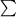:  A finite set of symbols, called the alphabet.

3.  Q x-->  Q  is the transition function.

4. q0 in Q is the start state,  and

5. F is a set of final states (or accept states).  F is a subset of Q.

```

The component-3 of the above definition implies that there is one outgoing transition from ever state for every symbol of the alphabet (sigma). Please note that the alphabet does not include the ^ symbol (which stands for the null string). F can be empty; that is, there may not be any final state in an FA as indicated in component-5 of the above definition. For a simple example of an FA or DFA, please visit this website.

The input to a finite automaton is a string of letters from the alphabet. The input is finite in length, that is read left to right one character at a time. Beginning in the start state, the machine reads a letter and makes a transition over and over until the string ends. If this process ends with the machine in an accept state, we say that the string is accepted. Otherwise the string is rejected. For an animation of a finite automaton, please visit this website.

We draw a Finite Automaton (FA) in the following manner: The machine’s states are represented by circles and transitions are represented by arrows. Every transition is marked by a symbol from the alphabet.  The start state is marked by a special short arrow that comes from nowhere, and the final states are marked by double circles. (For an explanation of     a*ba*, please click here.)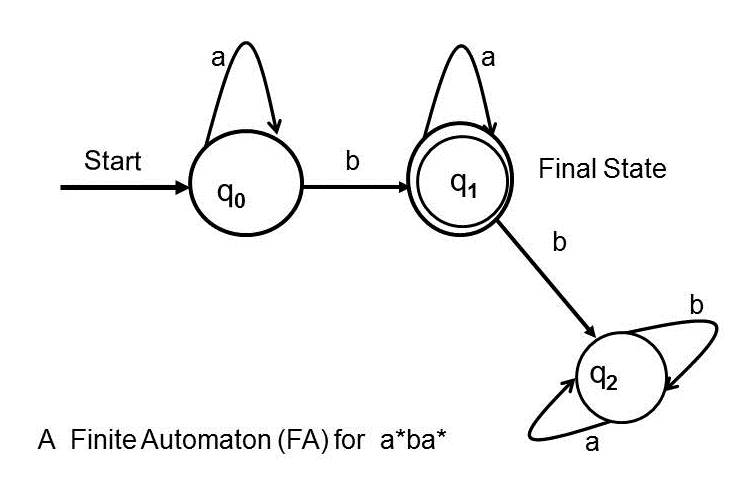Figure 1: A Finite Automaton (FA) for a*ba*

The finite automaton given above has one start state. Of course, every finite automaton has exactly one start state by definition.   The above finite automaton has one final state although finite automata are allowed to have  zero or more final states. The set of strings accepted by a finite automaton is referred to as the language accepted by the finite automaton (or the regular expression defined by the finite automaton).  The above finite automaton accepts the language  defined by  a*ba*.  The intuitive explanation is that the machine starts at  the start state and can take the loop transition marked by the symbol a  from the start state zero or more times and then take the arrow marked by b  and reach the final state where it can take the loop transition marked by a   as many time as it likes and finish at the final state marked in order to accept an input string. From the final state, if  the transition marked by b is taken then the machine will reach the non-final state that has two loops.  However,  it does not add to the language the machine accepts.  Because,  a finite automata’s accepted language is defined by tracing the paths from the start state to the final state(s). Paths ending in the non-final states do not contribute to the accepted language of the machine. The above notation for finite automata is given by M. Sipser (2012) Introduction to the Theory of Computation, 3rd Edition, Cengage Learning, and Hopcroft, Motwani & Ullman (2007) Introduction to Automata Theory, Languages, and Computation. Finite automata can be drawn with different notations. The above FA for a*ba* is drawn below in Figure 2 in the notation of Cohen, 1997, Introduction to Computer Theory. In Cohen's notation the start state is marked by a - sign and the final states are marked by a + sign.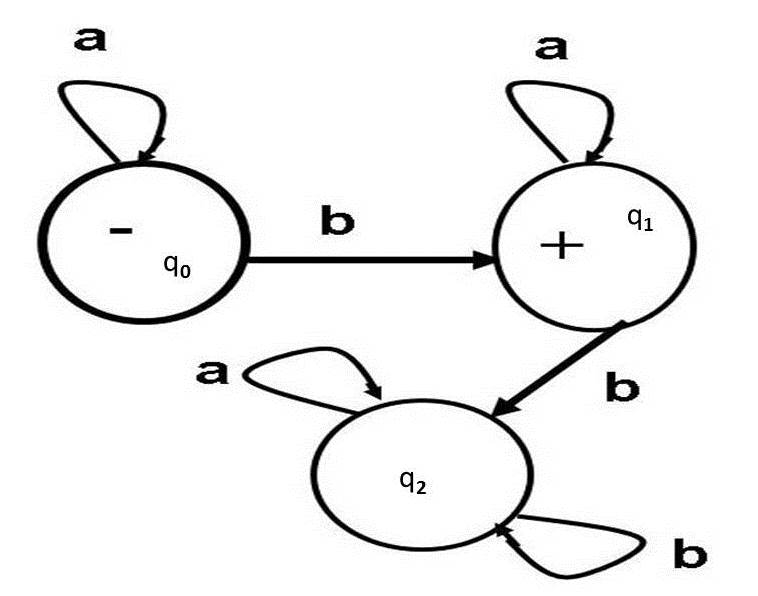Figure 2: A Finite Automaton (FA) for a*ba* in Cohen's (1997) notation

Please note that the terms Finite Automata (FA) and Deterministic Finite Automata (DFA) are used interchangeably; by FA one means DFA. Non-Deterministic Finite Automata (NFA) are different from DFA, by definition; NFA are explained later.

We draw another Finite Automaton (FA)  below which accepts every string from  ab*.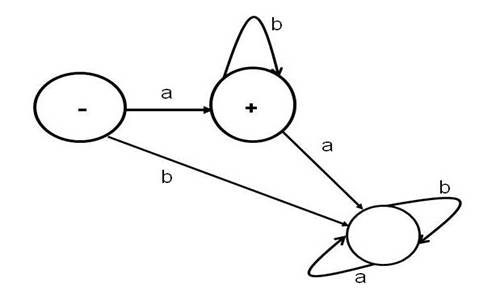A type of dynamic visualization of the above FA is given at: http://www.asethome.org/fa/. For a demonstration, please click here.

Please note that the Non-Deterministic Finite Automaton (NFA) for the same regular expression,  ab*, is given below which looks simpler than FA or DFA.  Why?  Because in order to meet the definition of DFA or FA, there should be one transition for every element offrom each state, whereas this restriction does not apply to NFA. .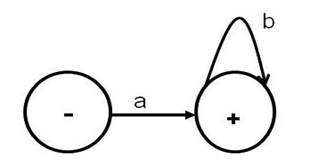We draw another FA or DFA which accepts all strings from  a*bb*aa*(ba*bb*aa*)*.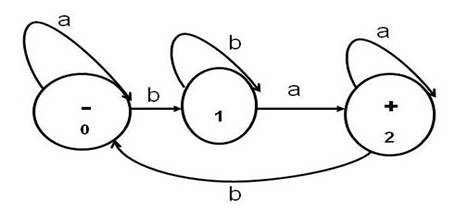As mentioned before, please note that some books show the start state with an incoming arrow that is not attached to any other state (instead of a sign). Accept states or final states are often drawn as double concentric circles in some books (instead of a  + sign). States are given names (0,1,2) to make discussions of the behavior of the machine easier. Transitions are shown as labeled arcs from one state to another or from a state back to itself. In the above, if the machine is in the start state 0 and it reads an a, the transition leaves state 0 and reenters state 0. If it reads a b from state 0, the machine enters state 1.

It is common for each state to have a transition leaving it for each letter of the alphabet. The above machine, when given the string bbabba will end up in state 2 and accept the string. If it reads string abbbab it ends up in state 0 and does not accept the string. We say that the machine rejects abbbab.

Note that the author uses a slightly different schema for drawing finite automata. He puts a minus sign in the start state and plus signs in the accept states.

The set of strings accepted by a finite automaton is referred to as the language accepted by the finite automaton. We might describe a finite automaton as a language recognizer whereas a regular expression is a language generator.

For each finite automaton there is a regular expression that defines the same language. Later we will learn an algorithm for determining the regular expression, but sometimes we can figure it out using our common sense. Look back at the above finite automaton. A regular expression corresponding to that machine is a*bb*aa*(ba*bb*aa*)*. Note that the portion before the parentheses moves you from the start state to the accept state. The portion in the parentheses moves from the accept state back to the accept state and this expression is "starred" because you can repeat this cycle as many times as necessary.

Another way to represent a finite automaton is with a transition table. Here is the table for the above machine. The rows correspond to states, the columns correspond to characters from the alphabet, and the cell contents correspond to the transitions of the machine.

 State a b 0 0 1 1 2 1 2 2 0

A drawing of a finite automaton is easier for a human to understand than a table, but implementing a machine with a computer program requires storing the finite automaton's transitions in a table.  There is not just one finite automaton for a given language. Typically it is possible to draw the finite automaton in any of several different ways.

There are languages for which it is not possible to draw any finite automaton. For example,  there is no FA for L3 =  { anbn: where n > 0  }.  Later we will show that any language that can be described by a regular expression can also be described by a finite automaton and vice versa.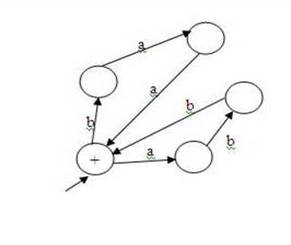A regular expression for the above machine is (baa + abb)*. Can you find a regular expression for this next one?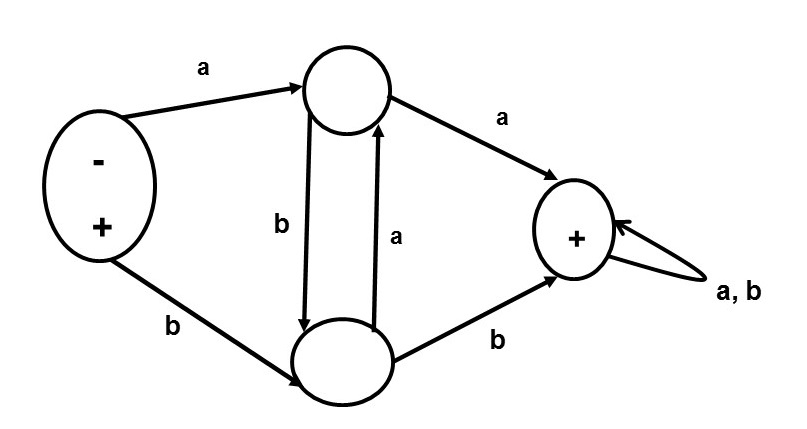If you try running some strings through the most recent machine you may notice that what it takes to get to the accept state is to see either two a's in a row or two b's. So the regular expression for the machine is (a+b)*(aa+bb)(a+b)*.

Let's try one more: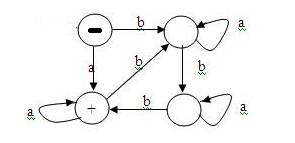Notice that this machine will accept any string consisting entirely of a's. It will also accept any string in which the number of b's is divisible by 3. Since the start state is not an accept state, the machine does not accept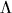. A regular expression for the machine is (aa* + ba*ba*ba*)(a + ba*ba*ba*)*.

How do you create a finite automaton that will accept any string with an odd number of a's? As the machine reads a's, you need to move back and forth between two states, one where the number of a's so far is even and one where the number is odd. Since we are not concerned with the number of b's, each state should have a self-transition for a b (a transition that leaves and enters the same state.) Which of the two states should be the start state? Since 0 is even and at the beginning we have seen 0 a's, the start state should be the state that corresponds to having seen an even number of a's. The other state, the one for having seen an odd number of a's, is an accept state. A machine that accepts strings with an even number of a's would look just like the one for odd a's except that the other state is the accept state.

What if we are concerned about the parity (oddness or evenness) of both a's and b's? For example, we might want to accept strings that contain an odd number of a's and an even number of b's. To figure out how many states we need we must ask how many different combinations of odd and even there are for two letters. The answer to this question is four: even/even, even/odd, odd/even, and odd/odd. The even/even state is the start state. Which states are accept states depends on the language we wish to accept. If we want strings that contain an odd number of a's and an even number of b's then we make the state odd/even be the only accept state. If we want strings in which the parity of a's matches the parity of b's then we make both even/even and odd/odd be accept states.

Here is one final example of a finite automaton. What strings are accepted by this one?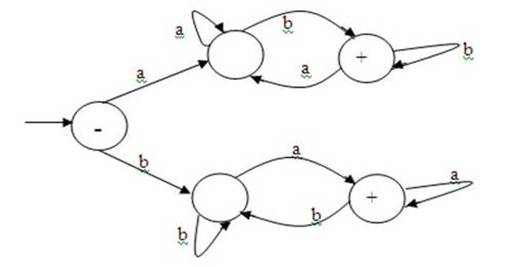There are two accept states in this machine. In order to get to the top one, the first letter of the string must be a and the last letter must be b. In order to get to the bottom one, the first letter must be b and the last one a. So this machine accepts strings that start and end with a different letter. There is no way to do this job with a single accept state. The machine must remember what the first letter was by taking one or the other of the transitions off of the start state. Once the machine has taken either the "high road" or the "low road" it cannot leave that portion of the machine or it would "forget" the first letter.

The  following Non-deterministic Finite Automaton would accept  any string from the regular expression  b*aba*b.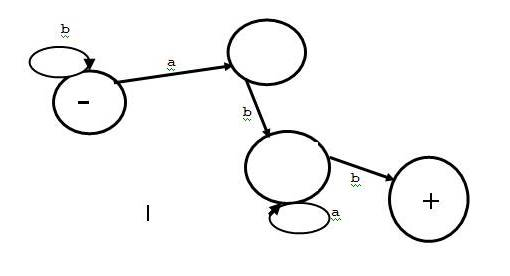```
Non-deterministic Finite Automata (NFA) are distinct from the
Deterministic FA or DFA (or FA) because NFA do not require one
outgoing transition from every state for each symbol of the alphabet.
(see Sipser (2005) page 48).
```

A nondeterministic finite automaton (NFA) is composed of 5 components:

```  1. Q:   A finite set of states.

2.:  A finite set of symbols, called the alphabet.

3.  Q xU ^   -->  Powerset(Q)  is the transition function.

4. q0 in Q is the start state,  and

5. F is a set of final states (or accept states).  F is a subset of Q.

```

It is to be noted that the component-3 of the above definition implies that null-transitions (that is, transitions with the null symbol, ^,) are also allowed in NFA. It is proven by Kleene that NFA are equivalent to  Deterministic FA. A DFA (Deterministic FA )for b*aba*b is given below: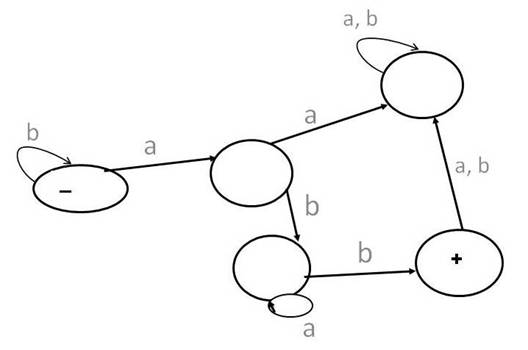Consider the following NFA (Non-deterministic Finite Automaton) for abcd*.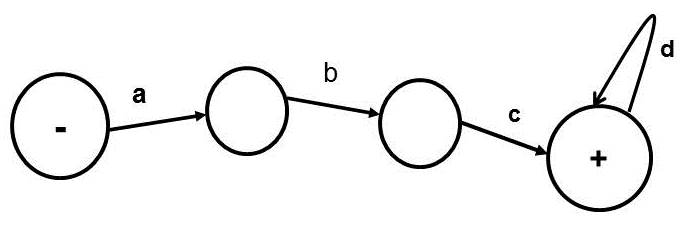A DFA (Deterministic Finite Automaton) for the same regular expression (abcd*) is given below: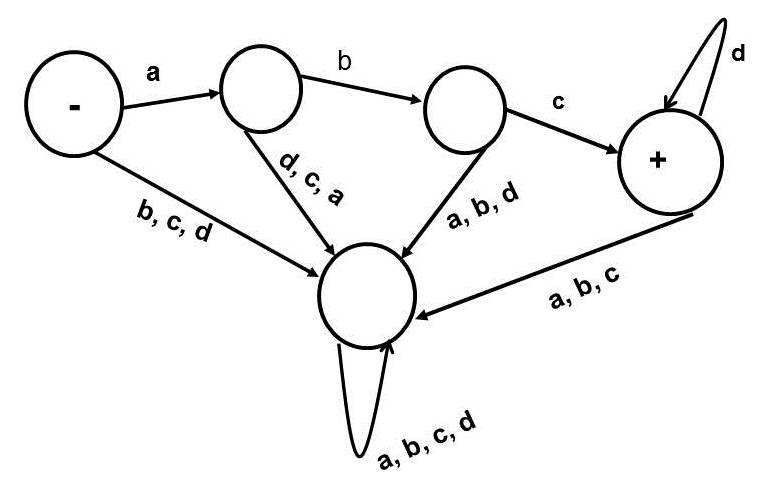Here is a recursive definition of the set of regular expressions over the alphabet:

1. Every element ofis a regular expression. ^ is a regular expression.
2. If R1 and R2 are regular expressions, then so are

R1R2, R1+R2, R1* and (R1).

1. Nothing else is a regular expression

Accourding to rule 2, concatenation, union, Kleene Star and parentheses can be used to create new regular expressions from old ones. Suppose= { a, b }, then a and b are regular expressions according to rule 1. According to rule 2, ab,     a+b,     a*     and     (a) are also regular expressions . Please note that a+b is often written as       a U b     (see Sipser, M. (2006) Introduction to the Theory of Computation , Course Technology.)

Consider the following example:= {1 }
1* = {^, 1, 11, 111, 1111, 11111, . . . }

The regular expression, 1*, includes an infinite set of strings given in {^, 1, 11, 111, 1111, 11111, . . . }. From the same alphabet consider the following two regular expressions:

11*     = {1, 11, 111, 1111, 11111, . . . }

11*1 = {11, 111, 1111, 11111, 111111, . . . }

Consider another example of regular expression given below:= {0, 1}
01* = {0, 01, 011, 0111, 01111, 011111, . . . }

Please note that the alphabet (sigma) is often not mentioned, if it is understood in the context. Thus, the following regular expression implies that the alphabet is {a, b}.

abba* = {abb, abba, abbaa, abbaaa, abbaaaa, . . . }

(See Cohen (1997) Introduction to Computer Theory, 2nd Edition, John Wiley & Sons, for details )

In this chapter we define a new type of finite state machine called a transition graph. These machines were invented in 1957 by John Myhill in order to simplify the proof of a theorem known as Kleene's Theorem.

A Transition Graph (TG) is basically a generalized nondeterministic finite automaton.  The following features of TG’s are emphasized:

1. We allow the labels on the transitions of a transition graph to be strings in addition to single characters (although we prefer single characters).
2. We allowas a transition label. This is interpreted as a "free move" from one state to another, a move that does not involve reading any input.
3. We allow the machine to read more than one character at a time.
4. There can be two transitions leaving a state that both have the same label.

Here is an example of a transition graph that illustrates some of the quirks of such machines. In this machine there are two different accepting paths that the machine may take on the string baab. There is no path at all for strings other than baab, so the string abb is rejected as are all strings other than baab.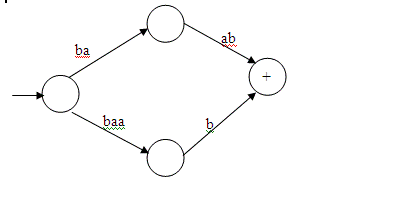If any path for a particular input leads to an accept state, we say the string is accepted. What if one path for string X leads to an accept state and one to a non-accept state? Then that string is accepted.

Self-Checking Exercises:  (You are encouraged to do these exercises. You are not required to submit them)

1. Construct a Finite Automaton that accepts only the word baa.

1. Construct a Transition Graph  that accepts only the word baa.

1. Build a  Transition Graph that accepts only those words that have more than 4 letters.